AI人工智能服务首页 > 科技动态 > 正文

## 聚类分析：创建，可视化以及可解释性

2021-01-18# 导读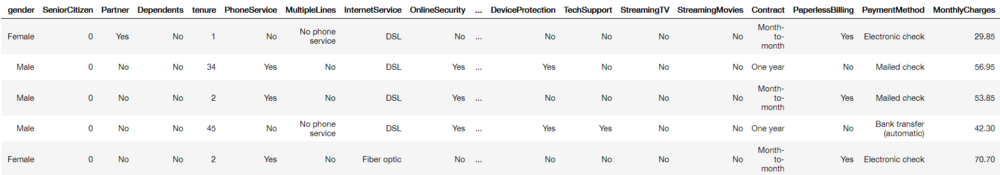# 聚类算法

k-Means聚类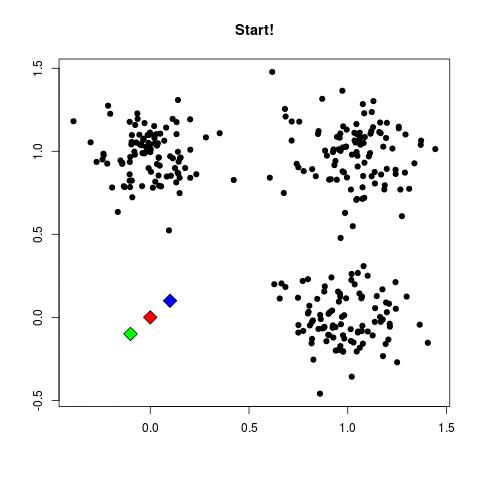```import seaborn as sns
import matplotlib.pyplot as plt
from sklearn.cluster import KMeans
scores = [KMeans(n_clusters=i+2).fit(df).inertia_ for i in range(10)]
sns.lineplot(np.arange(2, 12), scores)
plt.xlabel('Number of clusters')
plt.ylabel("Inertia")
plt.title("Inertia of k-Means versus number of clusters")```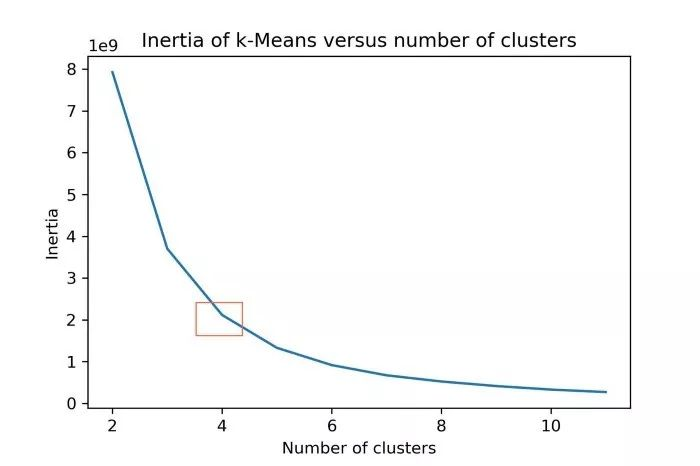```from sklearn import preprocessing
normalized_vectors = preprocessing.normalize(df)
scores = [KMeans(n_clusters=i+2).fit(normalized_vectors).inertia_ for i in range(10)]
sns.lineplot(np.arange(2, 12), scores)
plt.xlabel('Number of clusters')
plt.ylabel("Inertia")
plt.title("Inertia of Cosine k-Means versus number of clusters")
plt.savefig("intertia_cosine_kmeans.jpg", dpi=300)```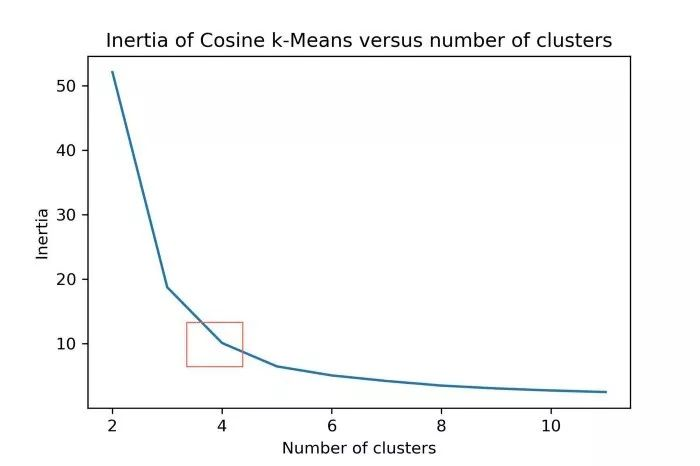k-Means在计算上非常昂贵。更快的替代方法是MiniBatchKMeans和BIRCH。这两种方法生成聚类的速度都更快，但是这些聚类的质量通常低于k-Means生成的聚类。

DBSCAN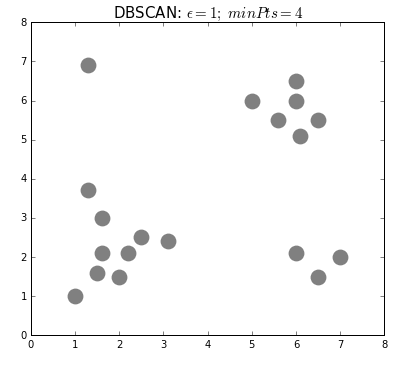DBSCAN的另一个替代方案是光学，它具有与DBSCAN类似的性能，但不需要显式地设置 eps。

# 评估聚类

```from sklearn.metrics import silhouette_score
# Prepare models
kmeans = KMeans(n_clusters=4).fit(df)
normalized_vectors = preprocessing.normalize(df)
normalized_kmeans = KMeans(n_clusters=4).fit(normalized_vectors)
min_samples = df.shape+1
dbscan = DBSCAN(eps=3.5, min_samples=min_samples).fit(df)
# Print results
print('kmeans: {}'.format(silhouette_score(df, kmeans.labels_, metric='euclidean')))
print('Cosine kmeans:{}'.format(silhouette_score(normalized_vectors,
normalized_kmeans.labels_,
metric='cosine')))
print('DBSCAN: {}'.format(silhouette_score(df, dbscan.labels_, metric='cosine')))```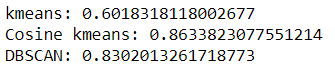# 可视化聚类

PCA

PCA的工作原理是利用正交变换将相关特征转换为一组线性无关特征的值。剩下的是包含最大可能方差的特征。

```tsne_3d_df = prepare_tsne(3, df, kmeans.labels_)
tsne_3d_df['normalized_kmeans'] = normalized_kmeans.labels_
tsne_3d_df['dbscan'] = dbscan.labels_
plot_animation(tsne_3d_df, 'kmeans', 'kmeans')
plot_animation(tsne_3d_df, 'normalized_kmeans', 'normalized_kmeans')
plot_animation(tsne_3d_df, 'dbscan', 'dbscan')```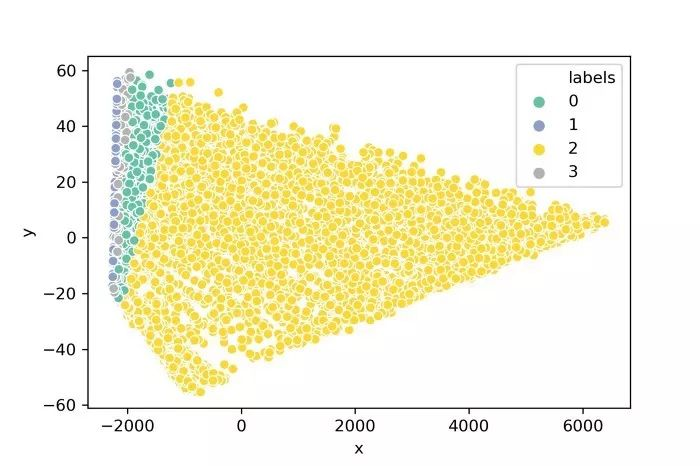t-SNE

t-SNE是一种可视化高维数据的算法。它使用点之间的局部关系来创建低维映射，从而捕获非线性结构。

```tsne_3d_df = prepare_tsne(3, df, kmeans.labels_)
tsne_3d_df['normalized_kmeans'] = normalized_kmeans.labels_
tsne_3d_df['dbscan'] = dbscan.labels_
plot_animation(tsne_3d_df, 'kmeans', 'kmeans')
plot_animation(tsne_3d_df, 'normalized_kmeans', 'normalized_kmeans')
plot_animation(tsne_3d_df, 'dbscan', 'dbscan')```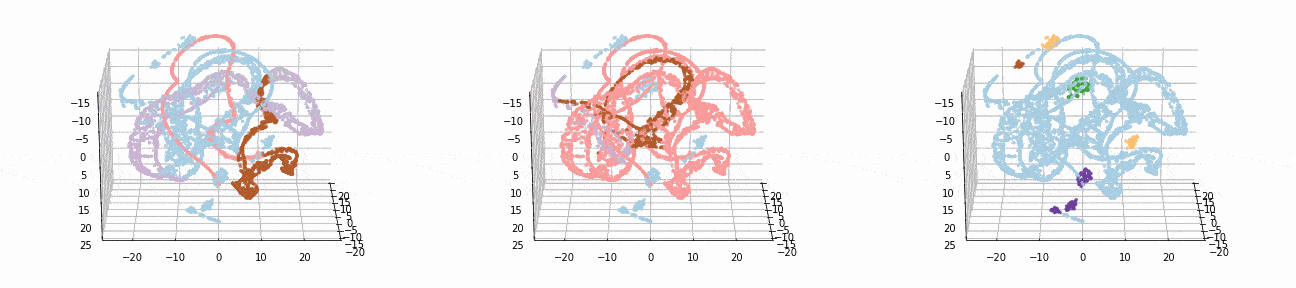Euclidean k-Means (LEFT), Cosine k-Means (MIDDLE), DBSCAN, (RIGHT)

t-SNE为数据提供了更直观的可视化表示。从动画中可以看出，cos k-Means和DBSCAN似乎都创建了逻辑聚类。

# 解释聚类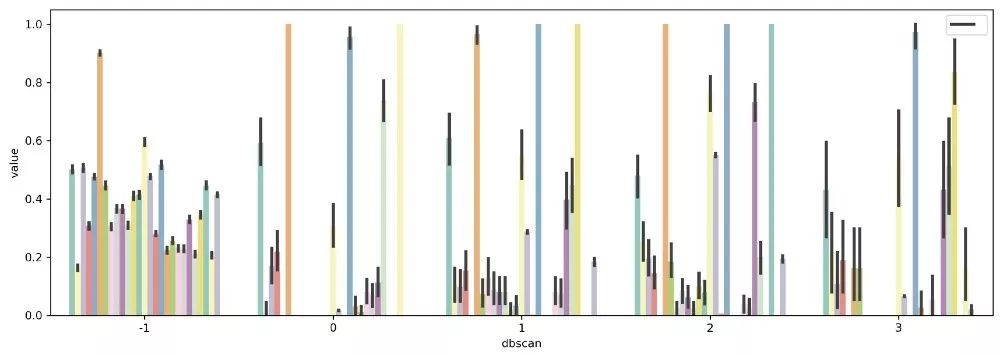```from sklearn.preprocessing import MinMaxScaler
scaler = MinMaxScaler()
df_scaled = pd.DataFrame(scaler.fit_transform(df))
df_scaled['dbscan'] = dbscan.labels_
df_mean = (df_scaled.loc[df_scaled.dbscan!=-1, :].groupby('dbscan').mean())```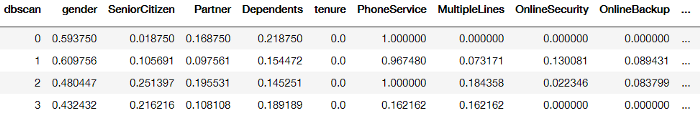DBSCAN生成的聚类中每个变量的均值

```results = pd.DataFrame(columns=['Variable', 'Var'])
for column in df_mean.columns[1:]:
results.loc[len(results), :] = [column, np.var(df_mean[column])]
selected_columns = list(results.sort_values('Var', ascending=False,).head(7).Variable.values) + ['dbscan']
tidy = df_scaled[selected_columns].melt(id_vars='dbscan')
sns.barplot(x='dbscan', y='value', hue='variable', data=tidy)```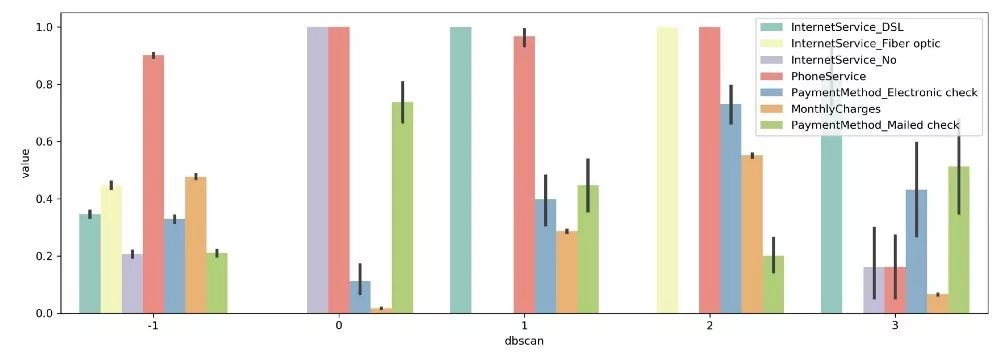：在比较变量时，我没有考虑标准差、偏度和峰度等重要因素。上面的方法只是选择变量的第一步。

```from sklearn.ensemble import RandomForestClassifier
X, y = df.iloc[:,:-1], df.iloc[:,-1]
clf = RandomForestClassifier(n_estimators=100).fit(X, y)
data = np.array([clf.feature_importances_, X.columns]).T
columns = list(pd.DataFrame(data, columns=['Importance', 'Feature'])
.sort_values("Importance", ascending=False)
tidy = df_scaled[columns+['dbscan']].melt(id_vars='dbscan')
sns.barplot(x='dbscan', y='value', hue='variable', data=tidy)```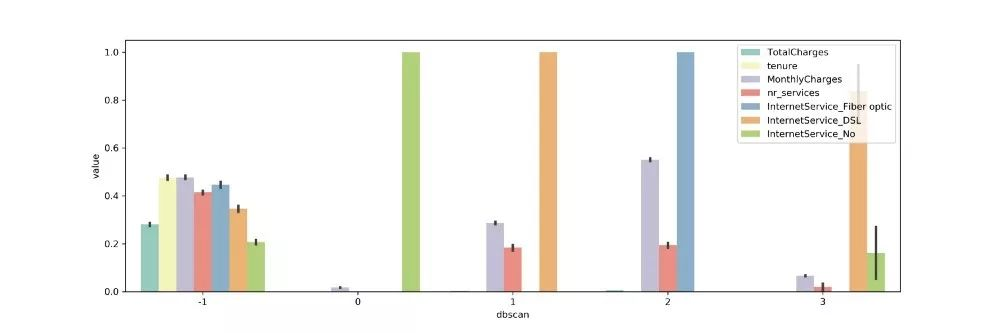• 北京市海淀区大钟寺13号华杰大厦B座3层306-310室
• 010-56181910

• 郑州市金水区紫荆山路5号
• 0371-60921991

• 石家庄市新华路294号盛安大厦5楼
• 18633018987